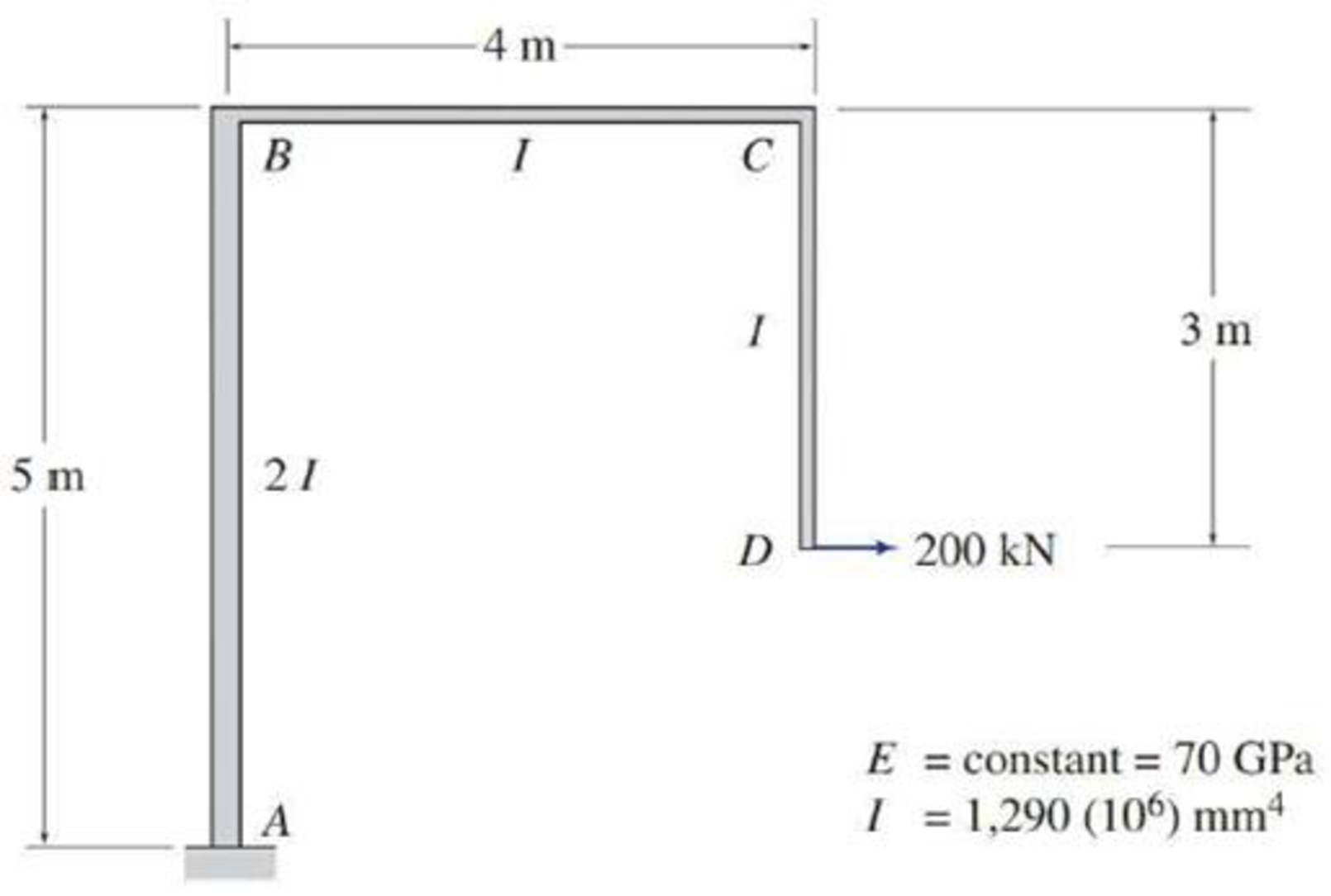# Use Castigliano’s second theorem to determine the rotation of joint D of the frame shown in Fig. P7.43. FIG. P7.43, P7.44, P7.67

#### Solutions

Chapter
Section
Chapter 7, Problem 67P
Textbook Problem
994 views

## Use Castigliano’s second theorem to determine the rotation of joint D of the frame shown in Fig. P7.43.FIG. P7.43, P7.44, P7.67

To determine

Find the rotation at joint D of the frame using Castigliano’s second theorem.

### Explanation of Solution

Given information:

The frame is given in the Figure.

The value of E is 70 GPa and I is 1,290×106mm4.

Apply the sign conventions for calculating reactions, forces and moments using the three equations of equilibrium as shown below.

• For summation of forces along x-direction is equal to zero (Fx=0), consider the forces acting towards right side as positive (+) and the forces acting towards left side as negative ().
• For summation of forces along y-direction is equal to zero (Fy=0), consider the upward force as positive (+) and the downward force as negative ().
• For summation of moment about a point is equal to zero (Matapoint=0), consider the clockwise moment as negative and the counter clockwise moment as positive.

Calculation:

Let apply a fictitious couple M¯ at joint D in the desired direction to find the slope.

The value of fictitious couple M¯ is zero.

Sketch the frame with fictitious couple M¯ as shown in Figure 1.

Let the equation for bending moment at distance x in terms of couple M¯ be M, the derivative of M with respect to M¯ is MM¯.

Find the reactions at the supports:

Summation of moments about A is equal to 0.

MA=0MA200(2)M¯=0MA=400+M¯

Summation of forces along x-direction is equal to 0.

+Fx=0Ax+200=0Ax=200kN

Sketch the member end forces of the frame as shown in Figure 2.

Find the equations for M and MM¯ for the 3 segments of the frame as shown in Table 1.

 Segment x-coordinate M ∂M∂M¯ Origin Limits (m) AB A 0−5 −(400+M¯)+200x −1 BC B 0−4 600−M¯ −1 DC D 0−3 −200x+M¯ 1

The expression for slope at D using Castigliano’s second theorem (θD) is shown as follows:

ΔD=0L(MM¯)MEIdx (1)

Here, L is the length of the beam.

Moment of inertia of span AB is 2I, moment of inertia of span BC is I, and moment of inertia of span DC is I.

Rearrange Equation (1) for the limits 05, 04, and 03 as follows.

θD=1EI[1205(MM¯)Mdx+04(MM¯)Mdx+03(MM¯)Mdx]

Substitute the value of fictitious couple M¯ as zero in the Column 4 of Table 1

### Still sussing out bartleby?

Check out a sample textbook solution.

See a sample solution

#### The Solution to Your Study Problems

Bartleby provides explanations to thousands of textbook problems written by our experts, many with advanced degrees!

Get Started

Find more solutions based on key concepts
Give examples of properties that engineers consider when selecting a material for a product or an application.

Engineering Fundamentals: An Introduction to Engineering (MindTap Course List)

What are the three major axes used during CNC mill programming?

Precision Machining Technology (MindTap Course List)

Identify several specific characteristics of intelligent behavior.

Principles of Information Systems (MindTap Course List)

How can you synchronize the calendar on your mobile device with the calendar on your home computer?

Enhanced Discovering Computers 2017 (Shelly Cashman Series) (MindTap Course List)

Create the ERD to show the relationships among EMPLOYEE, BENEFIT, JOB, and PLAN.

Database Systems: Design, Implementation, & Management

What is drill-down analysis and how is it used?

Fundamentals of Information Systems

How would you explode DFDs?

Systems Analysis and Design (Shelly Cashman Series) (MindTap Course List)

If your motherboard supports ECC DDR3 memory, can you substitute non-ECC DDR3 memory?

A+ Guide to Hardware (Standalone Book) (MindTap Course List)

The AWS Visual Inspection Criteria allows one small porosity per inches of weld.

Welding: Principles and Applications (MindTap Course List)